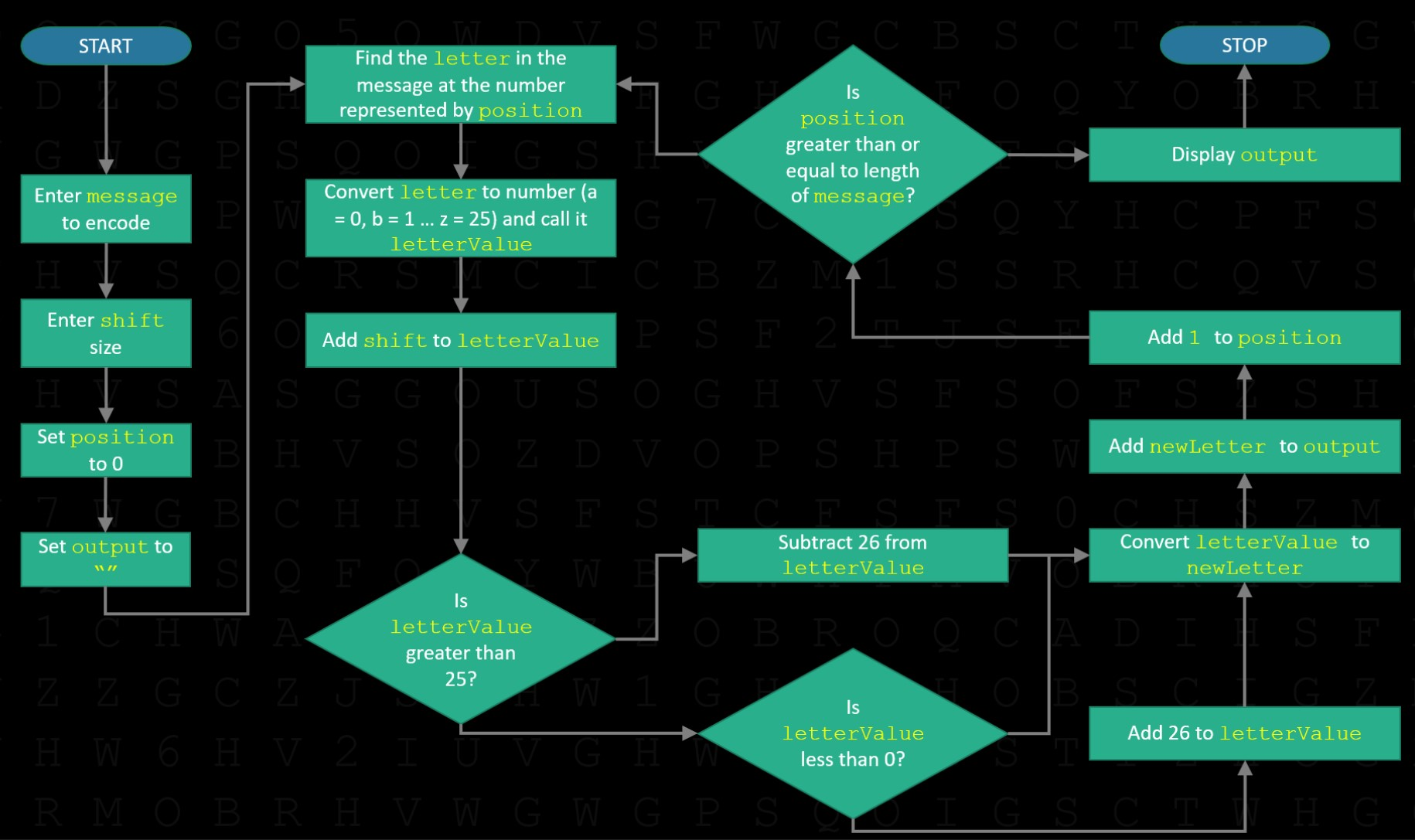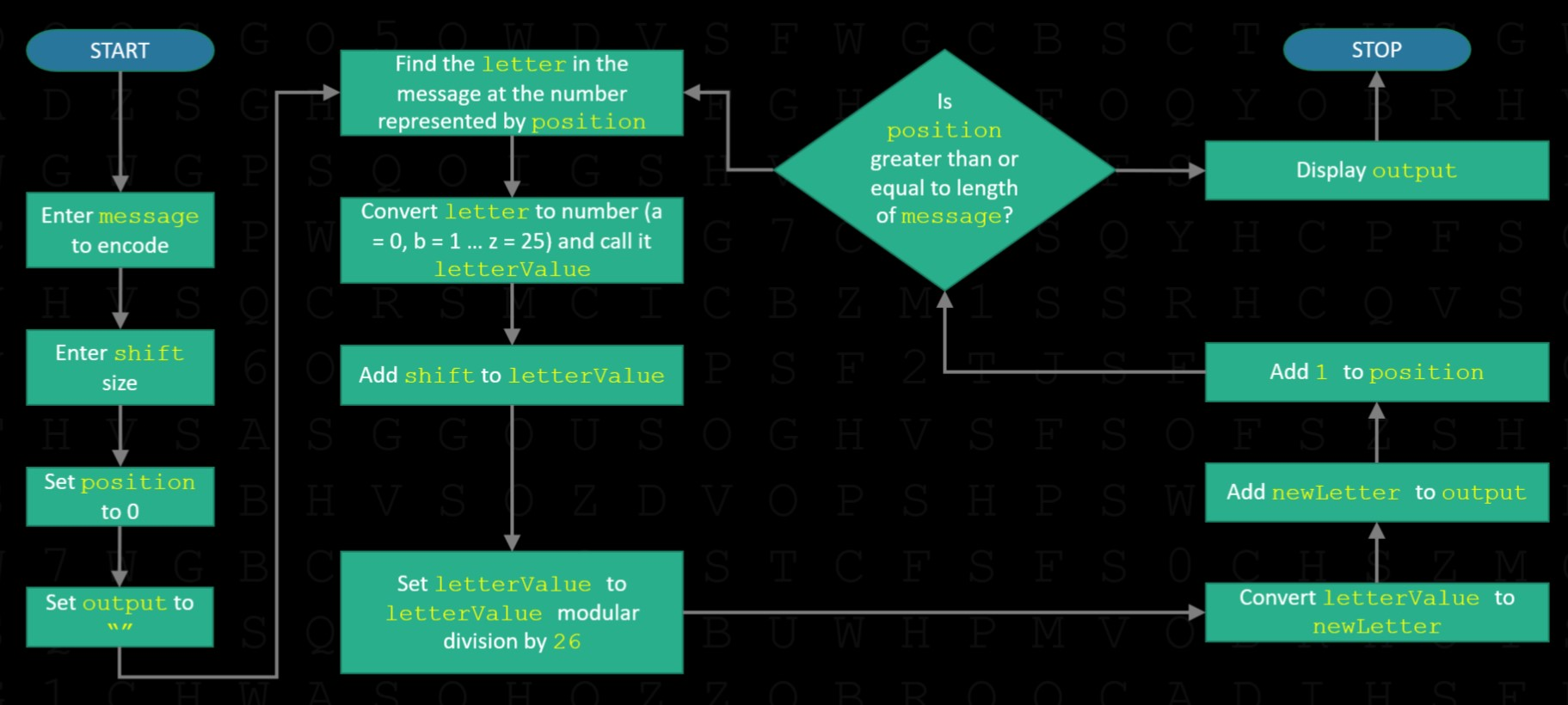# Caesar Cipher – Algorithm

An initial attempt to turn the Caesar cipher into code may look something like this:#### A problem with the algorithm

The chief problem with the algorithm above is that it only works for shift sizes between (and including) 0 and 25.

Let's say we are trying to encode the letter 'Z', which, as a number, is 25 (remember 'A' = 0, not 1). If a user now enters a shift size of 27, then the alogrithm will add 27 to 25 to get 52. The algorithm checks to see if this new 'letterValue' is greater than 25, which it is, and then subtracts 26 from it, leaving us with 26. But 26 is beyond the range of our alphabet, which starts at 0 (for 'A') and finishes at 25 (for 'Z').

A similar problem happens with negative shift sizes. Let's say we are trying to encode the letter 'A', which, as a number, is 0. If a user enters a shift size of -1, then the alogrithm will add -1 to 0 to get -1. The algorithm checks to see if this new 'letterValue' is greater than 25, which it is not, so it leaves it unchanged. But -1 is also beyond the range of our alphabet, which starts at 0 (for 'A') and finishes at 25 (for 'Z').

#### Improving the algorithm – modular division

There is a neat mathematical trick that solves both the problem of negative shift sizes and ones larger than 26, and it involves modular division.

Modular division gives the remainder whenever you divide two numbers. The symbol commonly used in many programming languages to denote modular division is the % sign. So 4 % 3 means '4 modular division by 3', or 'What is the remainder when you divide 4 by 3?'. The answer, of course, is 1. Other examples:

• 8 % 2 = 0
• 3 % 3 = 0
• 4 % 3 = 1
• 5 % 3 = 2
• 6 % 3 = 0
• 7 % 3 = 1
• 27 % 26 = 1
• 52 % 26 = 0
• 262 % 26 = 2
• -1 % 26 = 25
• -10 % 26 = 16
• -25 % 26 = 1
• -26 % 26 = 0
• -27 % 26 = 25

It does not matter what number we do modular division by 26 with, we always end up with an answer between and including 0 and 25, which is the range of our alphabet. We can use this to both streamline and improve our algorithm:Cipher Challenge competition    Leave feedback